# Knowledge Base

## Solution 34619: Finding the Limit of a Function on the TI-84 Plus CE and TI-84 Plus C Silver Edition.

### How can I find the limits on a TI-84 Plus CE and TI-84 Plus C Silver Edition?

While there is no built in function on the TI-84 Plus CE and TI-84 Plus C Silver Edition that can easily find the limits on the calculator, there is a work around. Using the example (x+1)/(x-1) let us find the limit as X approaches 3:

1) Press [Y=].
2) Press [( ] [x] [+]  [ )] [/] [( ] [x] [-]  [ )].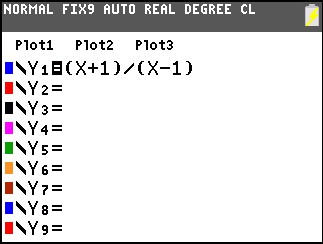3) Press [WINDOW] and enter the settings below.
4) Press [GRAPH] to display the graph.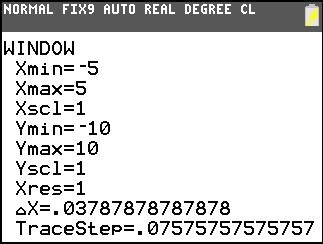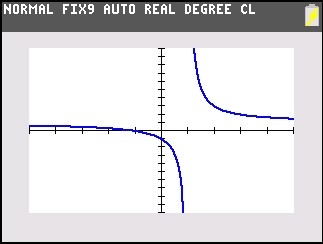5) Press [2nd] followed by [TBLSET].
6) Enter the settings below.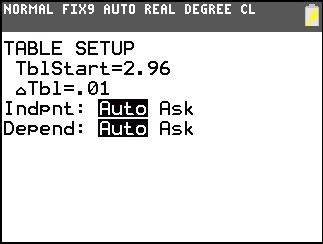7) Press [2nd] followed by [TABLE].
8) Looking at the numbers in the table as x approaches 3.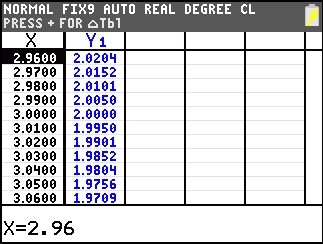This will give us the limit as X approaches 3, which is equal to 2.

Please see the TI-84 Plus CE and TI-84 Plus C Silver Edition guidebook for additional information.